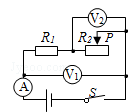$\text{A.}$ 电流表A示数变大 $\text{B.}$ 电压表V2示数变小 $\text{C.}$ 电压表$V_1$示数与 电压表$V_2$示数的差值变大 $\text{D.}$ 电压表V_1\$示数与电流表A示数的比值变大